Design of Experiments Guide > Prospective Sample Size and Power > Counts per Unit Calculator > Statistical Details for the Counts per Unit Calculator
Publication date: 08/13/2020

## Statistical Details for the Counts per Unit Calculator

Calculations for the counts per unit calculator are based on applying a normal approximation to the Poisson distribution. The test uses the following hypothesis: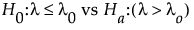The above hypothesis uses the following test statistic: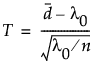where n is the sample size and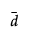is the mean number of defects per unit in the sample. We assume T to be approximately normally distributed. The power calculation is based on the distribution of T under the null and alternative hypotheses.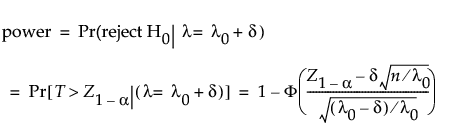where Φ() is the standard normal cumulative distribution function and Z1-p is the (1 - p)th quantile of the standard normal distribution.

Using 1 - β to denote the desired power to reject the null hypothesis, the sample size is calculated as follows: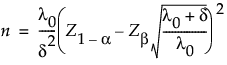Because an analytical solution for δ does not exist, numerical methods are used to solve for δ given power and n.